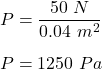A brick weighs 50.0 N, and measures 30.0 cm × 10.0 cm × 4.00 cm. What is the maximum pressure it can exert on a horizontal surface due to it

Question

A brick weighs 50.0 N, and measures 30.0 cm × 10.0 cm × 4.00 cm. What is the maximum pressure it can exert on a horizontal surface due to its weight?

in progress 0
5 months 2021-08-10T20:40:45+00:00 1 Answers 236 views 0

Pressure, P = 1250 Pa

Explanation:

Given that,

Weight of a brick, F = 50 N

Dimension of the brick is 30.0 cm × 10.0 cm × 4.00 cm

We need to find the maximum pressure it can exert on a horizontal surface due to its weight. Pressure is equal to the force acting per unit area. Pressure exerted is inversely proportional to the area of cross section. So, we need to minimize area. Taking to smaller dimensions.

A = 40 cm × 10 cm = 400 cm² = 0.04 m²

So,

Pressure,So, the maximum pressure of 1250 Pa it can exert on a horizontal surface.